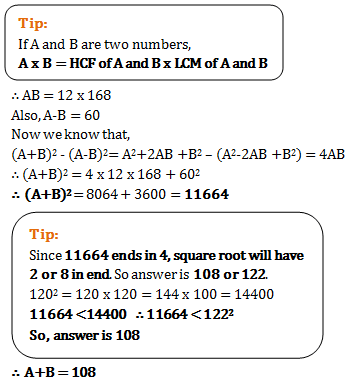# Problems on H.C.F and L.C.M - Quantitative Aptitude (MCQ) questions for Q. 29424

Q.  The least common multiple of two numbers is 168 and highest common factor of them is 12. If the difference between the numbers is 60, what is the sum of the numbers?
- Published on 12 Apr 17

a. 108
b. 96
c. 122
d. 144